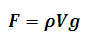# Archimedes Principle Calculator

This Calctown Calculator calculates the buoyant force exerted on a solid immersed in a liquid.

m3
kg/m3

#### Result

Nwhere

F = buoyant force

V = volume of solid immersed in the liquid

ρ = density of liquid

g = acceleration due to gravity